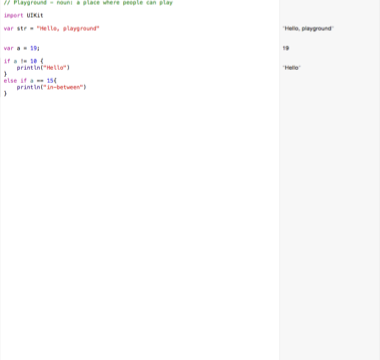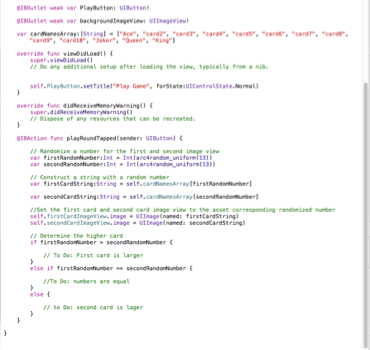# Xcode Swift Code:Introduction to IF Statements

Xcode Swift Code:Introduction to IF Statements

Today is an introduction using IF Statements with swift code in Xcode 6. In order to learn how created use statements I went back to Xcode playground.
An if statement is a condition with multiple outcomes. In order to for the statement to work, the conditions must be made. Depending on how the condition is met will determine the outcomes. Below in figure 1 is an example of how If Statements work.Figure 1:Experimenting the IF Conditions in Xcode Playground

Now that I understand how the function works, I head back to the card game project in Xcode 6. I then create the conditions in order to determine what will happen when the user presses the play button. Below in figure 2 is the implementation of the conditions.Figure 2: Implementing the IF conditions to the Card Game

The next step is to apply the scoring to the game in order to implement the set conditions.